# Nuclear Fusion Reactions

The nuclear fusion reaction that is of principal interest for the purposes of energy production in a magnetically confined thermonuclear plasma is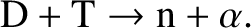(1.1)

Here, D denotes a deuterium nucleus (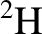), T denotes a tritium nucleus (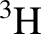), n denotes a neutron, and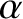denotes an alpha particle (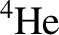). At achievable mean plasma temperatures (i.e., less than about 10 keV), a D-T fusion reaction has a cross-section that is approximately 100 times greater than that of a D-D fusion reaction (or a T-T fusion reaction) . (There is, unfortunately, no H-H fusion reaction.) For this reason, D-T fusion is considered to be more practical than D-D fusion, despite the fact that there is no natural source of tritium on the Earth. In fact, it is envisaged that D-T fusion reactors will breed the requisite tritium within a blanket that surrounds the plasma via nuclear reactions such as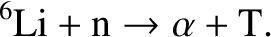(1.2)

Note that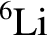makes up 7.6% of terrestrial lithium, which makes up about 0.002% of the Earth's crust.

A D-T fusion reaction liberates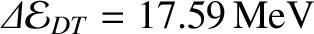(1.3)

of kinetic energy that is subsequently carried off by the fusion products . In a thermonuclear plasma, the momenta of the fusion products exceed those of the fusion reagents by many orders of magnitude, so the fusion reaction effectively takes place in the center of mass frame. Consequently, momentum and energy conservation require each product to carry off a fraction of the liberated energy that is inversely proportional to its mass (given that the products are moving non-relativistically) . Now, the masses of a neutron and an alpha particle are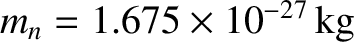and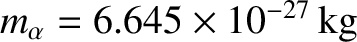, respectively. Hence, the kinetic energies of the neutron and the alpha particle generated by a D-T fusion reaction are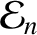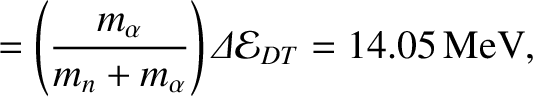(1.4)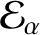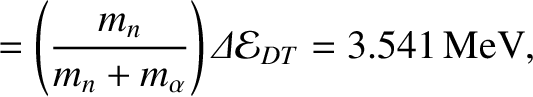(1.5)

respectively. In a magnetic confinement device, the alpha particles generated by D-T fusion reactions are confined by the magnetic field that permeates the plasma, and subsequently slow down and heat the deuterium and tritium nucleii, thereby, maintaining the nuclear fusion reactions. On the other hand, the neutrons generated by D-T fusion reactions exit the plasma, and are absorbed in the surrounding blanket. The energy absorbed by the blanket is extracted via a conventional heat exchanger, and used to generate electrical power. As has already been mentioned, the neutrons also breed tritium within the blanket, thereby, replacing the tritium burned up in the fusion reactions.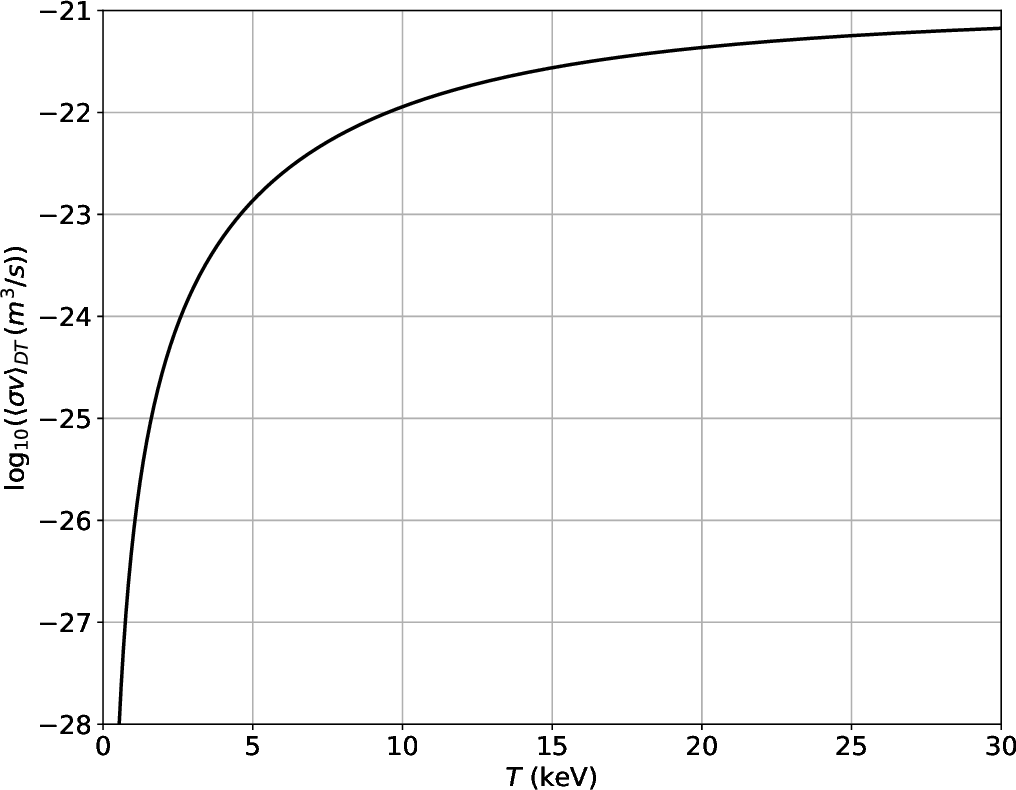Consider a thermonuclear plasma consisting, principally, of electrons, deuterium ions (that are fully stripped of electrons), and (fully-stripped) tritium ions. All three species are assumed to have have Maxwellian velocity distribution functions characterized by a common temperature,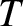. The rate of D-T fusion reactions occurring per unit volume within the plasma is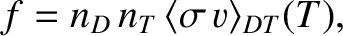(1.6)

where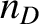is the deuteron number density,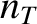the triton number density,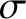the cross-section for D-T fusion reactions,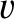the relative velocity of the reacting species, and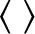denotes an average over the Maxwellian distributions of the reacting species. In the range of temperatures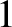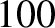keV, the D-T fusion reactivity,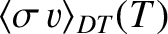, is accurately fitted by the following formula [2,4]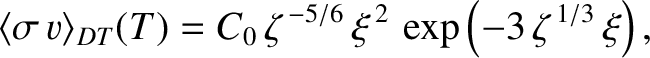(1.7)

where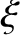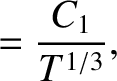(1.8)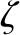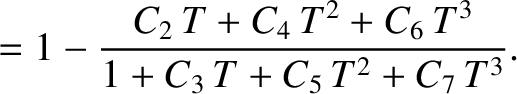(1.9)

Here,is measured in units of keV. The parameters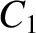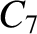are specified in Table 1.1. Figure 1.1 plotsfor a realistic range of plasma temperatures. It can be seen thatis a rapidly increasing function of increasing temperature, and that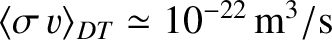when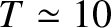keV.

Table: Parameters for formulae (1.7)–(1.9)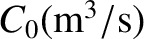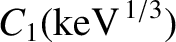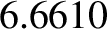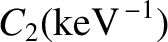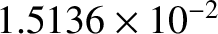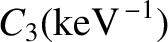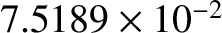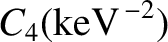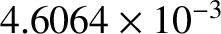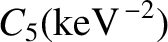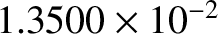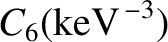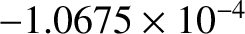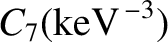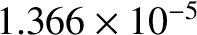.